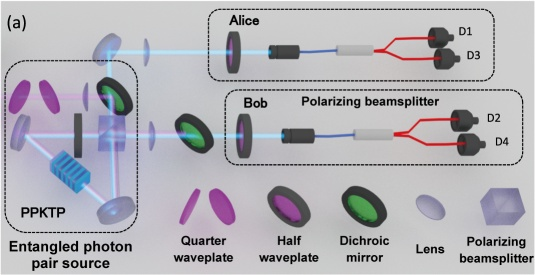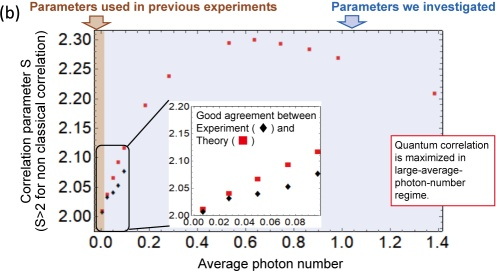Article from NICT NEWS 2021 No. 2（Vol. 486)

Researcher: Yoshiaki TSUJIMOTO

A quantum network is the ultimate network which aims to connect quantum devices such as quantum computers in order to derive the maximum functionality based on the principles of physics. This network uses a correlation specific to quantum mechanics called “quantum entanglement” as a resource to realize a protocol that is not attainable with conventional technology based on classical physics. This article explains the latest results of our research and development on the fast generation and application of entangled photon pairs that have such a quantum correlation, and a proof-of-principle experiment of the quantum protocol made possible using those entangled photon pairs.

### Creating a quantum network

The Internet has brought new values to society by connecting people and things. Similarly, the concept of a quantum network is a network that connects quantum devices such as quantum computers and quantum sensors. Distributed/blind quantum computing and ultra-precise clock synchronization and various other protocols using quantum networks have been proposed, and many other applications will emerge in the future. However, much technical development remains to attain the benefits that are expected of quantum networks. NICT has been conducting research and development on technologies for generating and controlling entangled photon pairs, which will provide a basic resource for quantum networks.

### Entanglement

Entanglement is a quantum correlation that cannot be explained by classical physics. Before exploring quantum entanglement, let’s look at a classical correlation by taking the polarization of photon pairs as an example. Here, we have a device that generates photon pairs polarized vertically or horizontally, which is selected at random. By distinguishing whether each photon is polarized vertically or horizontally using a polarization filter, a correlation in which one photon is vertically (horizontally) polarized and the other photon is also vertically (horizontally) polarized, should be observed. However, if we replace the filter to distinguish between clockwise and counter-clockwise circular polarization, no correlation is detected. A correlation obtained by only a specific polarization is called a classical correlation. On the other hand, if we use entangled photon pairs, both the measurement that distinguishes between vertical and horizontal polarization and the measurement that distinguishes between clockwise and counter-clockwise circular polarization will detect a correlation. This behavior cannot be explained by classical physics. A quantum network exploits such a quantum correlation.

### Development of a source of ultrafast entangled photon pairs

NICT has been developing a high-brightness/high-quality entangled photon pair source using non-linear optical effects called spontaneous parametric down-conversion (SPDC). Since SPDC generates photon pairs with a probability proportional to the intensity of a pump laser, it should be possible to increase the number of photon pairs by increasing the intensity of the pump laser. However, the higher the intensity of a pump laser, the larger the probability of generating two or more photon pairs in a single pulse, or an error event, which degrades entanglement. One way to avoid this trade-off is to raise the pulse repetition frequency of the pump laser. This decreases the energy per pulse, which increases the rate of generating entangled photon pairs without an increase in error events. We have succeeded in developing a new ultra-fast entangled photon pair source by combining a frequency comb source and a high-efficiency waveguide-type non-linear crystal (see Figure 1(a)). In this frequency comb source, the repetition frequency can be varied up to 50 GHz. As Figure 1(b) shows, the maximum detection rate of entangled photon pairs was 1.6 MHz, which was approximately a hundred times higher than the rate obtained in a previous experiment conducted by the Quantum ICT Advanced Development Center. In addition, as shown in Figure 1(c), we achieved high levels of fidelity, purity, and entanglement of formation (EoF), which represents the quality of quantum entanglement.
Figure 1
(a) Configuration of an ultra-fast entangled photon pair source: The entangled photon pairs are emitted from the waveguide-type nonlinear crystal. The pump beam is obtained by doubling the frequency of the high-speed optical pulses generated by electro-optical modulation (EOM).
(b) Relationship between the detection rate of entangled photon pairs and pumping power
(c) Relationship between the quality of entangled photon pairs and pumping power

### Experimental demonstration of quantum protocol

One of the protocols that use such entangled photon pairs as a resource is device-independent quantum key distribution (DIQKD). DIQKD is a next-generation QKD protocol that can obtain the secret key without any knowledge of the QKD device by monitoring the degree of quantum correlation between entangled photon pairs. The quantum correlation can be evaluated with the correlation parameter S, which is obtained from measurement, and if S exceeds 2, the security of the private key is guaranteed. However, when performing DIQKD using entangled photon pairs generated with SPDC, the optimal average photon number that maximizes S has not been clearly known. Using the experimental setup illustrated in Figure 2(a), we experimentally confirmed that S takes the maximum in a region of the average photon number that is significantly higher than conventionally thought (Figure 2(b)).Figure 2(a) Schematic of the experiment system to measure the correlation parameter, S, of entangled photon pairsFigure 2(b) Relationship between the average photon number of entangled photons and S
In addition, we experimentally demonstrated that long-distance DIQKD is possible by using a technique called entanglement swapping. The experimental setup shown in Figure 3(a) forms a quantum entanglement by generating two pairs of entangled photons and concatenating them. We applied entanglement swapping after adding the loss corresponding to a 50 km-long optical fiber to entangled photon pairs. The final state estimated from the result indicated that S > 2 (Figure 3(b)).